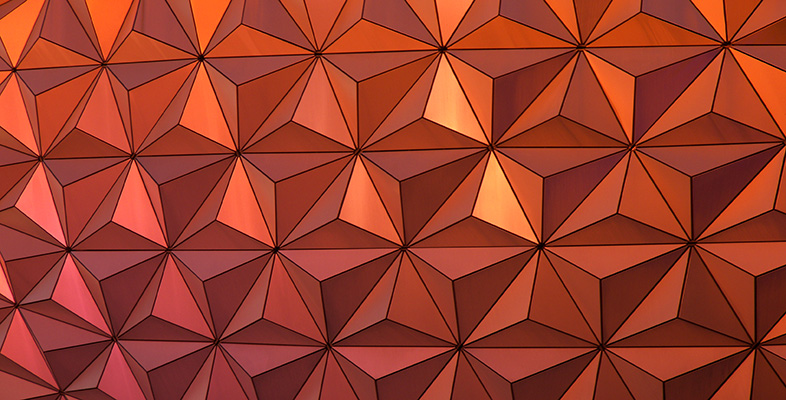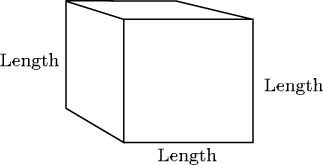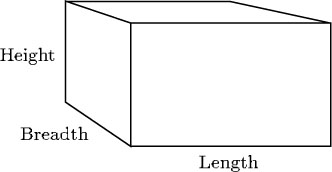Science, Maths & Technology

### Become an OU studentGeometry

Start this free course now. Just create an account and sign in. Enrol and complete the course for a free statement of participation or digital badge if available.

# 3.3 Volumes

What is a volume? The word usually refers to the amount of three-dimensional space that an object occupies. It is commonly measured in cubic centimetres (cm3) or cubic metres (m3).

A closely related idea is capacity; this is used to specify the volume of liquid or gas that a container can actually hold. You might refer to the volume of a brick and the capacity of a jug – but not vice versa. Note that a container with a particular volume will not necessarily have the same amount of capacity. For example, a toilet cistern will have a smaller capacity than its total volume because the overflow pipe makes the volume above the pipe outlet unusable. Some units are used only for capacity – examples are litre, gallon and pint; cubic centimetres and cubic metres can be used for either capacity or volume.

One of the simplest solid shapes is a cube; it has six identical square faces.

Volume of a cube = length × length × length = (length) 3A cuboid (or rectangular box) has 6 rectangular faces as shown below.

Volume of a rectangular box = length × breadth × heightThe length × breadth is the area of the bottom (or top) of the box, so an alternative formula is

volume of box = area of base × height.

The volume formula can also be written as

volume  = area of end face × length

or

volume = area of front face × breadth